A Source Book in Greek Science

Contents:
Author: Hero of Alexandria

Show Summary
Trigonometry

Hero of Alexandria, Metrica I. 8, III. 20, II. 125

I. 8. There is a general method whereby, if the three sides of any triangle are given, it is possible to find the area without finding the altitude.

For example, let the sides of the triangle be of 7, 8, and 9 units.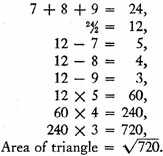1

Now since 720 has no rational square root, we shall obtain a very close approximation to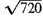as follows:

The nearest [perfect integral] square to 720 is 729.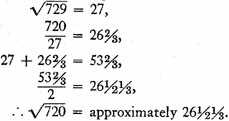For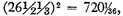the difference being only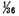of a unit.

Now if we desire that the difference be less than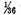we commence with the number just obtained,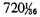instead of with 729, and by proceeding in the same way we shall find that the difference will be much less than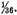2

III. 20. Let there be a pyramid, with base ABCD of any form, and vertex at point E, with side AE of 5 units. It is required to cut the pyramid by a plane parallel to the base in such a way that the pyramid cut off at the vertex is, let us say, four times the remaining solid. Let ZHTK be the section made by the cutting plane. Thus AZ will be a side of the solid ABCDZHTK.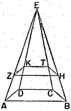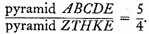Now pyramids are to each other as the cubes of homologous sides.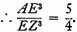But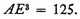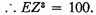We must therefore find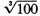by approximation.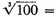very nearly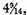as we shall show below.

Thus if EZ be taken as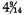and the pyramid be cut at point Z by a plane parallel to the base, the problem is solved.

The synthesis is as follows.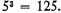Since the ratio of the two parts is 4:1, we have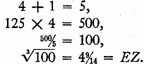We shall now show how to find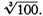Take the cubic numbers nearest to 100, both greater and less, i.e., 125 and 64.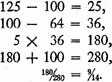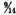to the cube root of the smaller cubic number  we have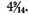This will be the approximation to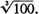1

II. 12. Let it be required to measure the segment of a sphere, having a base of diameter 12 and height 2.1

Now Archimedes proves that the ratio of a segment of a sphere to a cone with same base and equal height is equal to the ratio of the sum of the radius of the sphere and the height of the remaining segment, to the height of the remaining segment.2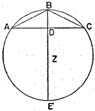Let the segment in question be ABC, with altitude BD. Let Z be the center of the sphere.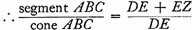That is,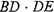is given.

But since BD is given, DE is also given, as is the whole of BE.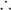EZ is given, and also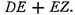But since DE is given, the ratio of the cone, with base equal to the diameter of circle AC and height BD, to the segment of the sphere is also given.The segment of the sphere is given.

We shall on the basis of this analysis proceed as follows: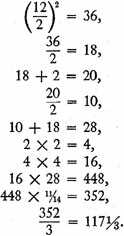This will be the volume of the segment.

1 This is an application of the so-called Heronian formula,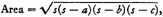where a, b, and c are the sides and s half the perimeter. The geometrical proof is given both in the Metrica and in the Dioptra of Hero, but there is strong evidence that we owe the formula to Archimedes.

2 The procedure is as follows:

Let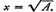If x1 is the first approximation, i.e., the square root of the nearest perfect square to A,

Let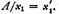Now, the second approximation is the arithmetic mean of x1 and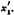(1) That is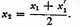Similarly let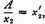(2) and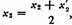and so on.

Hero, in the example given, does not go beyond equation (1). If the process is repeated we get, from equation (2),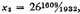of which the square is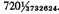For a discussion of this method in connection with Archimedes’ approximation to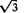(p. 61, above), see T. L. Heath, History of Greek Mathematics II. 325.

1 Precisely what general formula is applied in this procedure is not entirely clear. It has been suggested that the formula is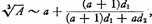where a3 and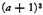are the nearest perfect cubes below and above A, and d1 and d2 the differences between A and a3, and A and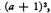respectively. For a discussion see T. L. Heath, op. cit., II. 341.

1 The reference evidently is to a segment of one base.

2 Archimedes, On the Sphere and the Cylinder II, Prop. 2, Corollary.

Contents:

### Related Resources

None available for this document.

Title: A Source Book in Greek Science

Select an option:

## Email Options

Title: A Source Book in Greek Science

Select an option: